# Two Floor Temperature Sensor

Need a sweater when you're downstairs but a Hawaiian shirt when you're upstairs? This two floor temperature sensor will help out your outfit

BeginnerShowcase (no instructions)2 hours567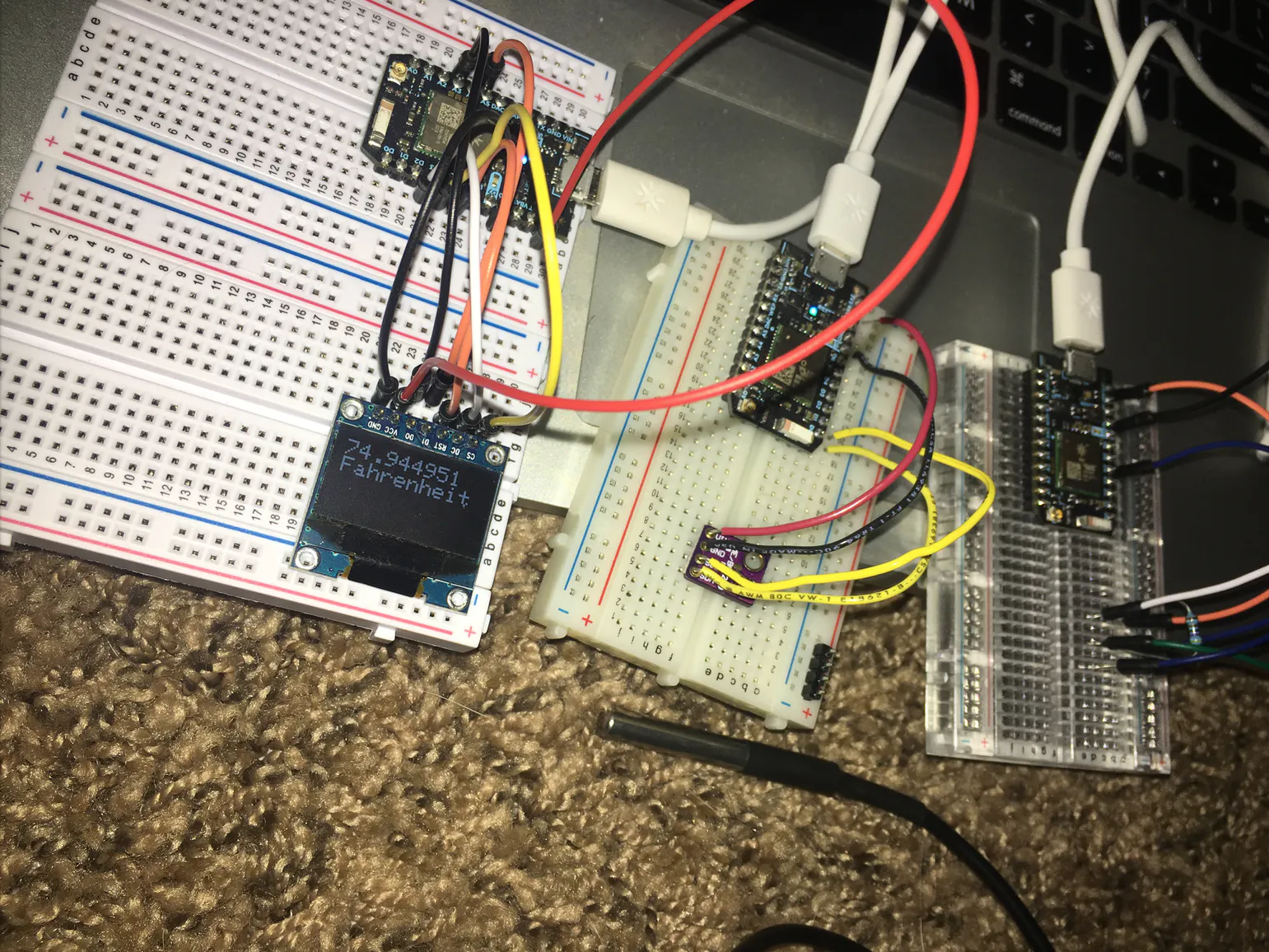## Things used in this project

### Hardware componentsParticle Photon
×3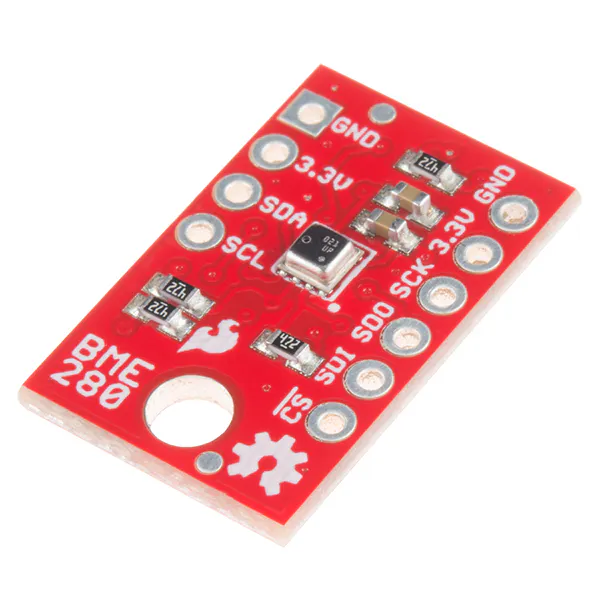SparkFun Atmospheric Sensor Breakout - BME280
×1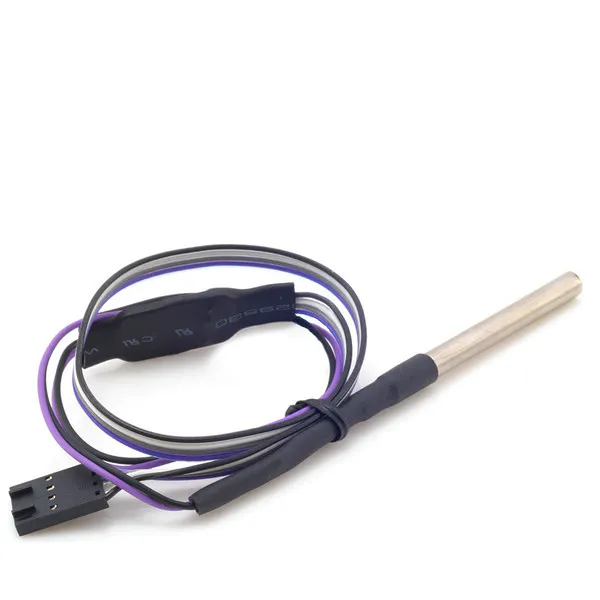Modulo Temperature Probe
×1
 OLED Display Screen
×1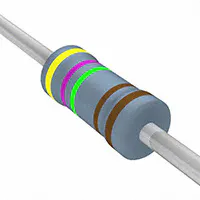Resistor 4.75k ohm
×1Jumper wires (generic)
×1×1

### Software apps and online services

 Particle.IoThingSpeak API

## Schematics

### Floor 1 Temperature Sensor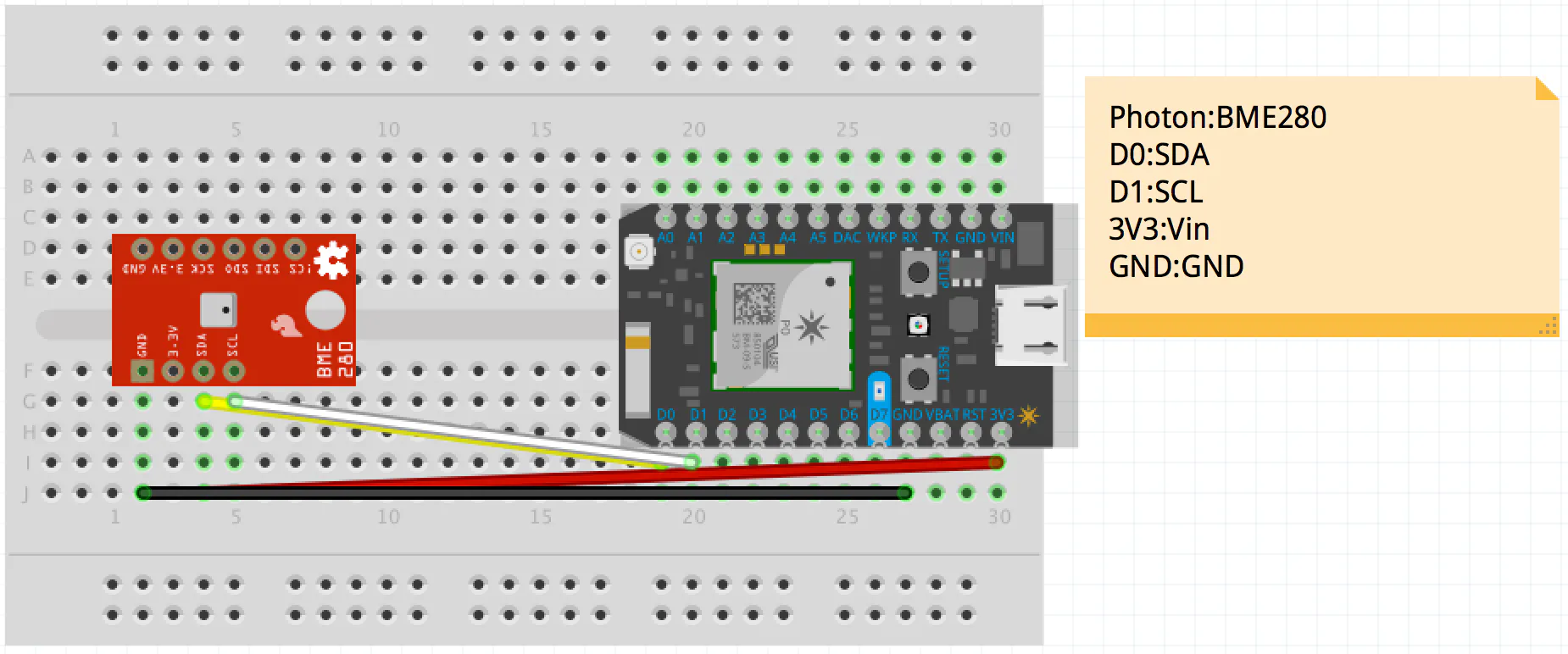### Floor 2 Temperature Sensor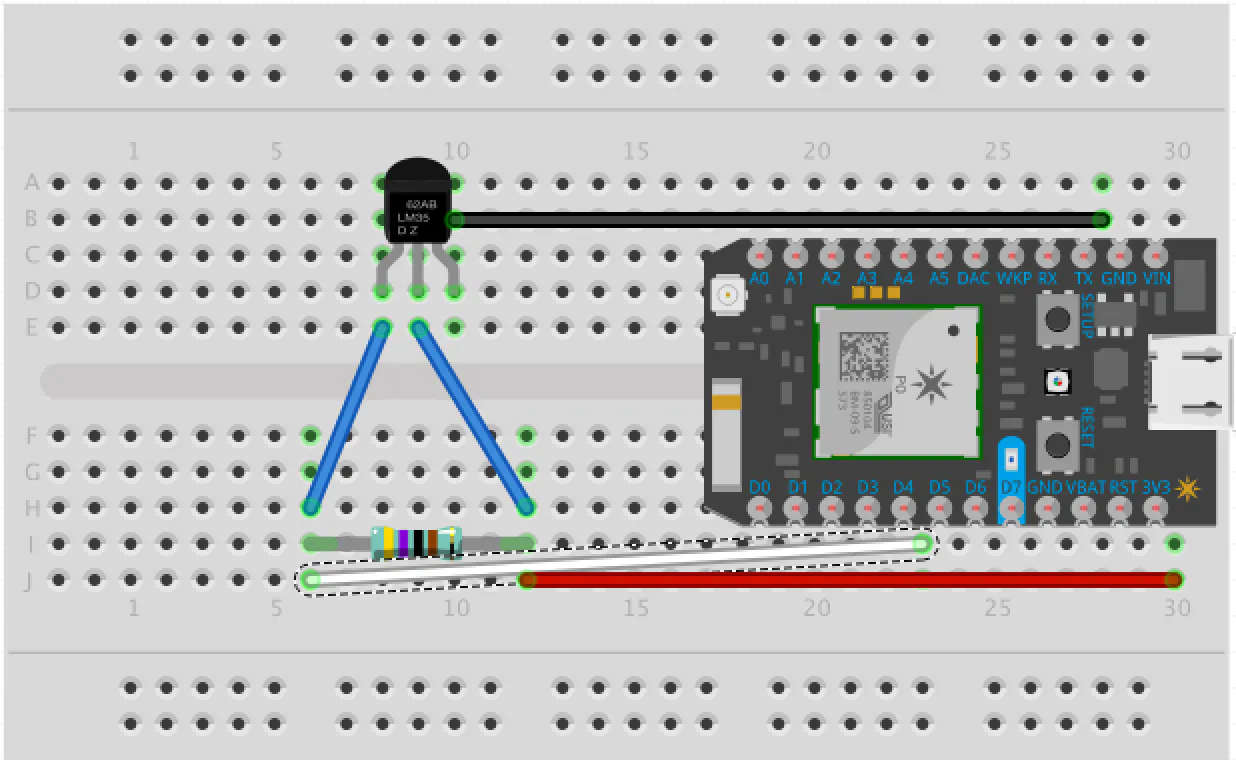### Display Screen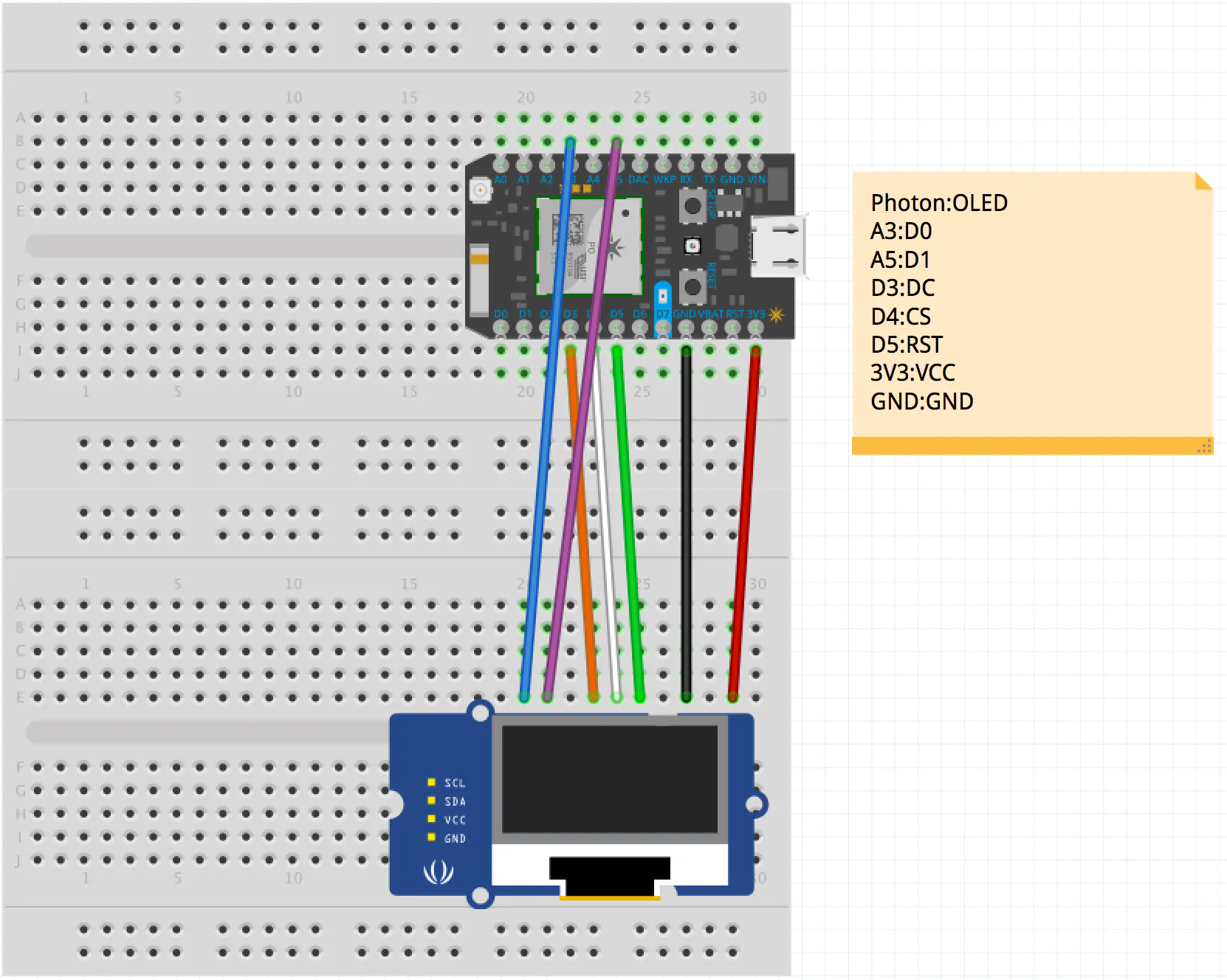## Code

### Floor 1 Temperature Sensor

C/C++
This uses the BME280 as the temperature sensor that is placed in the ground floor of my home.
```// Distributed with a free-will license.
// Use it any way you want, profit or free, provided it fits in the licenses of its associated works.
// BME280
// This code is designed to work with the BME280_I2CS I2C Mini Module available from ControlEverything.com.
// https://www.controleverything.com/content/Humidity?sku=BME280_I2CS#tabs-0-product_tabset-2

#include <application.h>
#include <spark_wiring_i2c.h>

// BME280 I2C address is 0x76(108)

#define publish_delay 16000
unsigned int lastPublish = 0;

double cTemp = 0, fTemp = 0, pressure = 0, humidity = 0;
void setup()
{
// Set variable
Particle.variable("i2cdevice", "BME280");
Particle.variable("cTemp", cTemp);
Particle.variable("fTemp", fTemp);
Particle.variable("pressure", pressure);
Particle.variable("humidity", humidity);

// Initialise I2C communication as MASTER
Wire.begin();
// Initialise Serial communication, set baud rate = 9600
Serial.begin(9600);
delay(300);

}

void loop()
{

unsigned int b1;
unsigned int data;
int dig_H1 = 0;
for(int i = 0; i < 24; i++)
{
// Start I2C Transmission
// Select data register
Wire.write((136+i));
// Stop I2C Transmission
Wire.endTransmission();

// Request 1 byte of data

// Read 24 bytes of data
if(Wire.available() == 1)
{
}
}

// Convert the data
// temp coefficents
int dig_T1 = (b1 & 0xff) + ((b1 & 0xff) * 256);
int dig_T2 = b1 + (b1 * 256);
int dig_T3 = b1 + (b1 * 256);

// pressure coefficents
int dig_P1 = (b1 & 0xff) + ((b1 & 0xff ) * 256);
int dig_P2 = b1 + (b1 * 256);
int dig_P3 = b1 + (b1 * 256);
int dig_P4 = b1 + (b1 * 256);
int dig_P5 = b1 + (b1 * 256);
int dig_P6 = b1 + (b1 * 256);
int dig_P7 = b1 + (b1 * 256);
int dig_P8 = b1 + (b1 * 256);
int dig_P9 = b1 + (b1 * 256);

for(int i = 0; i < 7; i++)
{
// Start I2C Transmission
// Select data register
Wire.write((225+i));
// Stop I2C Transmission
Wire.endTransmission();

// Request 1 byte of data

// Read 7 bytes of data
if(Wire.available() == 1)
{
}
}

// Convert the data
// humidity coefficents
int dig_H2 = b1 + (b1 * 256);
int dig_H3 = b1 & 0xFF ;
int dig_H4 = (b1 * 16) + (b1 & 0xF);
int dig_H5 = (b1 / 16) + (b1 * 16);
int dig_H6 = b1;

// Start I2C Transmission
// Select data register
Wire.write(161);
// Stop I2C Transmission
Wire.endTransmission();

// Request 1 byte of data

// Read 1 byte of data
if(Wire.available() == 1)
{
}

// Start I2C Transmission
// Select control humidity register
Wire.write(0xF2);
// Humidity over sampling rate = 1
Wire.write(0x01);
// Stop I2C Transmission
Wire.endTransmission();

// Start I2C Transmission
// Select control measurement register
Wire.write(0xF4);
// Normal mode, temp and pressure over sampling rate = 1
Wire.write(0x27);
// Stop I2C Transmission
Wire.endTransmission();

// Start I2C Transmission
// Select config register
Wire.write(0xF5);
// Stand_by time = 1000ms
Wire.write(0xA0);
// Stop I2C Transmission
Wire.endTransmission();

for(int i = 0; i < 8; i++)
{
// Start I2C Transmission
// Select data register
Wire.write((247+i));
// Stop I2C Transmission
Wire.endTransmission();

// Request 1 byte of data

// Read 8 bytes of data
if(Wire.available() == 1)
{
}
}

// Convert pressure and temperature data to 19-bits
long adc_p = (((long)(data & 0xFF) * 65536) + ((long)(data & 0xFF) * 256) + (long)(data & 0xF0)) / 16;
long adc_t = (((long)(data & 0xFF) * 65536) + ((long)(data & 0xFF) * 256) + (long)(data & 0xF0)) / 16;
// Convert the humidity data
long adc_h = ((long)(data & 0xFF) * 256 + (long)(data & 0xFF));

// Temperature offset calculations
double var1 = (((double)adc_t) / 16384.0 - ((double)dig_T1) / 1024.0) * ((double)dig_T2);
double var2 = ((((double)adc_t) / 131072.0 - ((double)dig_T1) / 8192.0) *
double t_fine = (long)(var1 + var2);
double cTemp = (var1 + var2) / 5475.0;
double fTemp = cTemp * 1.8 + 32;

/*

// Pressure offset calculations
var1 = ((double)t_fine / 2.0) - 64000.0;
var2 = var1 * var1 * ((double)dig_P6) / 32768.0;
var2 = var2 + var1 * ((double)dig_P5) * 2.0;
var2 = (var2 / 4.0) + (((double)dig_P4) * 65536.0);
var1 = (((double) dig_P3) * var1 * var1 / 524288.0 + ((double) dig_P2) * var1) / 524288.0;
var1 = (1.0 + var1 / 32768.0) * ((double)dig_P1);
double p = 1048576.0 - (double)adc_p;
p = (p - (var2 / 4096.0)) * 6250.0 / var1;
var1 = ((double) dig_P9) * p * p / 2147483648.0;
var2 = p * ((double) dig_P8) / 32768.0;
double pressure = (p + (var1 + var2 + ((double)dig_P7)) / 16.0) / 100 ;

// Humidity offset calculations
double var_H = (((double)t_fine) - 76800.0);
var_H = (adc_h - (dig_H4 * 64.0 + dig_H5 / 16384.0 * var_H)) * (dig_H2 / 65536.0 * (1.0 + dig_H6 / 67108864.0 * var_H * (1.0 + dig_H3 / 67108864.0 * var_H)));
double humidity = var_H * (1.0 -  dig_H1 * var_H / 524288.0);
if(humidity > 100.0)
{
humidity = 100.0;
}
else if(humidity < 0.0)
{
humidity = 0.0;
}

*/

// Output data to dashboard
Particle.publish("cTempHafBer", String(cTemp));
Particle.publish("fTempHafBer", String(fTemp));
// Particle.publish("Pressure : ", String(pressure));
// Particle.publish("Relative Humidity : ", String(humidity));
delay(10000);

unsigned long now = millis();
if ((now - lastPublish) < publish_delay) {
return;
}

Particle.publish("thingSpeakWrite_A0", "{ \"1\": \"" + String(fTemp) + "\", \"k\": \"XXXXXXXXXXXXXXXX\" }", 60, PRIVATE);
lastPublish = now;

};

void tempHandler(const char *event, const char *data){

Particle.publish(String(event), String(data));

};
```

### Floor 2 Temperature Sensor

C/C++
This uses the temperature sensor probe that is placed in the upstairs to retrieve data
```// This #include statement was automatically added by the Particle IDE.
#include <OneWire.h>

/************************************************************************
This sketch reads the temperature from a 1-Wire device and then publishes
to the Particle cloud. From there, IFTTT can be used to log the date,
here: https://docs.particle.io/tutorials/topics/maker-kit

This sketch is the same as the example from the OneWire library, but
with the addition of three lines at the end to publish the data to the
cloud.

Use this sketch to read the temperature from 1-Wire devices
you have attached to your Particle device (core, p0, p1, photon, electron)

Temperature is read from: DS18S20, DS18B20, DS1822, DS2438

Expanding on the enumeration process in the address scanner, this example
reads the temperature and outputs it from known device types as it scans.

I/O setup:
These made it easy to just 'plug in' my 18B20 (note that a bare TO-92
sensor may read higher than it should if it's right next to the Photon)

D3 - 1-wire ground, or just use regular pin and comment out below.
D4 - 1-wire signal, 2K-10K resistor to D5 (3v3)
D5 - 1-wire power, ditto ground comment.

A pull-up resistor is required on the signal line. The spec calls for a 4.7K.
I have used 1K-10K depending on the bus configuration and what I had out on the
bench. If you are powering the device, they all work. If you are using parisidic
power it gets more picky about the value.
************************************************************************/

OneWire ds = OneWire(D4);  // 1-wire signal on pin D4

unsigned long lastUpdate = 0;

float lastTemp;

#define publish_delay 16000
unsigned int lastPublish = 0;

void setup() {
Serial.begin(9600);
// Set up 'power' pins, comment out if not used!
pinMode(D3, OUTPUT);
pinMode(D5, OUTPUT);
digitalWrite(D3, LOW);
digitalWrite(D5, HIGH);
}

// up to here, it is the same as the address acanner
// we need a few more variables for this example

void loop(void) {
byte i;
byte present = 0;
byte type_s;
byte data;
float celsius, fahrenheit;

Serial.println();
ds.reset_search();
delay(250);
return;
}

// The order is changed a bit in this example
// first the returned address is printed

Serial.print("ROM =");
for( i = 0; i < 8; i++) {
Serial.write(' ');
}

// second the CRC is checked, on fail,

Serial.println("CRC is not valid!");
return;
}
Serial.println();

// we have a good address at this point
// what kind of chip do we have?
// we will set a type_s value for known types or just return

// the first ROM byte indicates which chip
case 0x10:
Serial.println("  Chip = DS1820/DS18S20");
type_s = 1;
break;
case 0x28:
Serial.println("  Chip = DS18B20");
type_s = 0;
break;
case 0x22:
Serial.println("  Chip = DS1822");
type_s = 0;
break;
case 0x26:
Serial.println("  Chip = DS2438");
type_s = 2;
break;
default:
Serial.println("Unknown device type.");
return;
}

// this device has temp so let's read it

ds.reset();               // first clear the 1-wire bus
ds.select(addr);          // now select the device we just found
// ds.write(0x44, 1);     // tell it to start a conversion, with parasite power on at the end
ds.write(0x44, 0);        // or start conversion in powered mode (bus finishes low)

// just wait a second while the conversion takes place
// different chips have different conversion times, check the specs, 1 sec is worse case + 250ms
// you could also communicate with other devices if you like but you would need

delay(1000);     // maybe 750ms is enough, maybe not, wait 1 sec for conversion

// we might do a ds.depower() (parasite) here, but the reset will take care of it.

// first make sure current values are in the scratch pad

present = ds.reset();
ds.write(0xB8,0);         // Recall Memory 0
ds.write(0x00,0);         // Recall Memory 0

present = ds.reset();
if (type_s == 2) {
ds.write(0x00,0);       // The DS2438 needs a page# to read
}

// transfer and print the values

Serial.print("  Data = ");
Serial.print(present, HEX);
Serial.print(" ");
for ( i = 0; i < 9; i++) {           // we need 9 bytes
Serial.print(data[i], HEX);
Serial.print(" ");
}
Serial.print(" CRC=");
Serial.print(OneWire::crc8(data, 8), HEX);
Serial.println();

// Convert the data to actual temperature
// because the result is a 16 bit signed integer, it should
// be stored to an "int16_t" type, which is always 16 bits
// even when compiled on a 32 bit processor.
int16_t raw = (data << 8) | data;
if (type_s == 2) raw = (data << 8) | data;
byte cfg = (data & 0x60);

switch (type_s) {
case 1:
raw = raw << 3; // 9 bit resolution default
if (data == 0x10) {
// "count remain" gives full 12 bit resolution
raw = (raw & 0xFFF0) + 12 - data;
}
celsius = (float)raw * 0.0625;
break;
case 0:
// at lower res, the low bits are undefined, so let's zero them
if (cfg == 0x00) raw = raw & ~7;  // 9 bit resolution, 93.75 ms
if (cfg == 0x20) raw = raw & ~3; // 10 bit res, 187.5 ms
if (cfg == 0x40) raw = raw & ~1; // 11 bit res, 375 ms
// default is 12 bit resolution, 750 ms conversion time
celsius = (float)raw * 0.0625;
break;

case 2:
data = (data >> 3) & 0x1f;
if (data > 127) {
celsius = (float)data - ((float)data * .03125);
}else{
celsius = (float)data + ((float)data * .03125);
}
}

// remove random errors
if((((celsius <= 0 && celsius > -1) && lastTemp > 5)) || celsius > 125) {
celsius = lastTemp;
}

fahrenheit = celsius * 1.8 + 32.0;
lastTemp = celsius;
Serial.print("  Temperature = ");
Serial.print(celsius);
Serial.print(" Celsius, ");
Serial.print(fahrenheit);
Serial.println(" Fahrenheit");

// now that we have the readings, we can publish them to the cloud
String temperature = String(fahrenheit); // store temp in "temperature" string
Particle.publish("TempHafBer", temperature, PUBLIC); // publish to cloud
delay(10000); // 10 second delay

unsigned long now = millis();
if ((now - lastPublish) < publish_delay) {
return;
}

Particle.publish("thingSpeakWrite_A0", "{ \"1\": \"" + String(fahrenheit) + "\", \"k\": \"XXXXXXXXXXXXXXXX\" }", 60, PRIVATE);
lastPublish = now;
}
```

### Display Screen

C/C++
Using the OLED, this display will alternate between the temperature readings from both floor 1 and floor 2
```// This #include statement was automatically added by the Particle IDE.

/*********************************************************************
This is an example for our Monochrome OLEDs based on SSD1306 drivers

Pick one up today in the adafruit shop!

This example is for a 128x64 size display using SPI to communicate
4 or 5 pins are required to interface

Adafruit invests time and resources providing this open source code,

All text above, and the splash screen must be included in any redistribution
*********************************************************************/

/* Uncomment this block to use hardware SPI
// If using software SPI (the default case):
#define OLED_MOSI   D0
#define OLED_CLK    D1
#define OLED_DC     D2
#define OLED_CS     D3
#define OLED_RESET  D4
Adafruit_SSD1306 display(OLED_MOSI, OLED_CLK, OLED_DC, OLED_RESET, OLED_CS);
*/

// use hardware SPI
#define OLED_DC     D3
#define OLED_CS     D4
#define OLED_RESET  D5

#define NUMFLAKES 10
#define XPOS 0
#define YPOS 1
#define DELTAY 2

#define LOGO16_GLCD_HEIGHT 16
#define LOGO16_GLCD_WIDTH  16

static const unsigned char logo16_glcd_bmp[] =
{ 0B00000000, 0B11000000,
0B00000001, 0B11000000,
0B00000001, 0B11000000,
0B00000011, 0B11100000,
0B11110011, 0B11100000,
0B11111110, 0B11111000,
0B01111110, 0B11111111,
0B00110011, 0B10011111,
0B00011111, 0B11111100,
0B00001101, 0B01110000,
0B00011011, 0B10100000,
0B00111111, 0B11100000,
0B00111111, 0B11110000,
0B01111100, 0B11110000,
0B01110000, 0B01110000,
0B00000000, 0B00110000 };

#if (SSD1306_LCDHEIGHT != 64)
#endif

//Get Temperature data from cloud
void tempF(const char *fTempHafBer, const char *dataF)
{
// display the temperature
display.setTextSize(2);
display.setTextColor(WHITE);
display.setCursor(0,0);
display.printlnf("%s Fahrenheit\n", dataF, 3);
display.display();
display.clearDisplay(); // clears the screen and buffer

}

void setup()   {
Serial.begin(9600);

// by default, we'll generate the high voltage from the 3.3v line internally! (neat!)
display.begin(SSD1306_SWITCHCAPVCC);
// init done
display.display(); // show splashscreen
delay(100);
display.clearDisplay();  // clears the screen and buffer

// Subscribe to tempearture variable
Particle.subscribe("fTempHafBer", tempF);
Particle.subscribe("TempHafBer", tempF);

Particle.publish("Collecting Temperature");

}
```

## Credits

### Eric Beresid

1 project • 0 followers

### Alex Hafner

1 project • 0 followers